bug sack filling up - WoWInterface
01-23-15, 06:12 PM   #1
Linds
A Kobold Labourer
Join Date: Dec 2014
Posts: 1
bug sack filling up

I have no idea what this means. Im not very techy at all. This stuff has been spamming my BugSack all day though. mostly while farming Naxx but there have been times in other places and have been happening both in and out of combat. Ive been using Carbonite for many years and have no intention of letting these bugs make me stop using it, just wanted to report it in case its something simply repairable.

Copied directly from BugSsck
49x Carbonite\NxMap.lua:6493: attempt to perform arithmetic on a nil value
Carbonite\NxMap.lua:6493: in function `UpdateOverlay'
Carbonite\NxMap.lua:6324: in function `UpdateZones'
Carbonite\NxMap.lua:4574: in function `Update'
Carbonite\NxMap.lua:3820: in function <Carbonite\NxMap.lua:3735>

Locals:
self = <table> {
MiniBlks = 6
IconScale = 3
}
}
MapPosY = -3731.4456300003
MMScalesC = <table> {
}
LOpts = <table> {
}
Guide = <table> {
}
PlyrLastDir = 89.999999316755
WorldHotspots = <table> {
}
}
EffScale = 0.75442045927048
Zone = 0
WorldAlpha = 0
StartupShown = true
ScaleDraw = 0.45431674872011
MoveLastX = 2751.9411351221
PlyrDir = 89.999999316755
PlyrSpeedCalcTime = 282652.646
MapPosYDraw = -3732.8220241361
PlyrSpeed = 0
MapScale = 0.045340992886238
CurMapBG = false
BGIncNum = 0
ViewSavedData = <table> {
}
MapW = 273.51992797852
MMGathererUpdateDelay = 1
LClickTime = 0
MouseEnabled = true
ButAutoScaleOn = <table> {
}
MapH = 205.0998840332
MMScales = <table> {
}
MapPosX = 2751.9411351221
BackgndAlphaTarget = 0.4
MoveDir = 180
BGGrowBars = true
ArrowScroll = 0.65
H = 141
Tick = 12614
IconNIFrms = <table> {
}
LastDungeonLevel = 0
BaseScale = 1
ToolBar = <table> {
}
MoveLastY = -3731.4456300003
}
DotPartyScale = 1
DotPalScale = 1
QuestAlpha = 0.3
CurWorldUpdateOverlayNum = 1
Scale = 0.45431674872011
TextFrm = <unnamed> {
}
BackgndAlpha = 0.4
ArrowPulse = 1
}
MapId = -1
MMOwn = false
Size1 = 0.99414058935418
ShowUnexplored = false
Arch = <unnamed> {
}
}
DotRaidScale = 1
}
StepTime = -15
PlyrSpeedX = 2751.9411351221
PlyrFrm = <unnamed> {
}
ScaleDrawW = 2.2011074934331
IconStaticFrms = <table> {
}
UpdateTrackingDelay = 0
IconNavScale = 1
MiniFrms = <table> {
}
InCombat = false
Win = <table> {
}
InstanceId = false
RealScale = 0.45431674872011
Data = <table> {
}
TrackDir = false
WorldHotspotTipStr = "Northrend, |cffffff00Dragonblight (71-80)
"
}
CurOverlays = <table> {
}
LocTipFStrs = <table> {
}
TrackPlyrs = <table> {
}
CurOverlaysTexFolder = "dragonblight"
}
TextScFrm = <unnamed> {
}
Targets = <table> {
}
}
W = 150
QuestWin = <unnamed> {
}
WorldHotspotsCity = <table> {
}
TrackDistYd = 29.802349645349
TrackName = "Wyrmrest Temple, Dragonblight"
CurWorldUpdateMapId = 488
Tracking = <table> {
}
ArchAlpha = 0.3
PlyrY = -3731.4456300003
TargetNextUniqueId = 1
TrackETA = false
TextFStrs = <table> {
}
Scrolling = false
}
PlyrRZY = 51.292562484741
ContFrms = <table> {
}
LocTipFrm = NxMapTip {
}
TileFrms = <table> {
}
ScaleW = 2.2011074934331
BackgndAlphaFull = 1
CurOpts = <table> {
}
}
TitleH = 0
IconFrms = <table> {
}
PlyrSpeedY = -3731.4456300003
Cont = 90
MapIndex = 1
MMOwnedFrms = <table> {
}
Level = 9
PlyrRZX = 87.134426832199
KillShow = false
MMChkDelay = 32
NeedWorldUpdate = false
MapPosXDraw = 2752.2482908499
MapsDrawnOrder = <table> {
}
Frm = <unnamed> {
}
}
UpdateTargetDelay = 0
DotZoneScale = 1
PlyrX = 2751.9411351221
}
mapId = -1
winfo = <table> {
1 = 1
2 = 0
3 = 0
}
s = 4
freeOrScale = true

**after clearing BugSack and doing one more Naxx run... bugged every time i threw an attack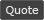WoWInterface » bug sack filling up

 Thread Tools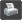Show Printable Version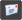Email this Page Display Modes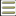Switch to Linear Mode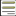Switch to Hybrid Mode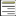Threaded Mode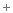Posting Rules You may not post new threads You may not post replies You may not post attachments You may not edit your posts vB code is On Smilies are On [IMG] code is On HTML code is Off